# numpy.bitwise_and¶

numpy.bitwise_and(x1, x2[, out])

Compute bit-wise AND of two arrays, element-wise.

When calculating the bit-wise AND between two elements, x and y, each element is first converted to its binary representation (which works just like the decimal system, only now we’re using 2 instead of 10):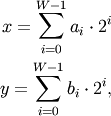where W is the bit-width of the type (i.e., 8 for a byte or uint8), and each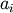and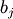is either 0 or 1. For example, 13 is represented as 00001101, which translates to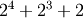.

The bit-wise operator is the result of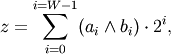where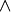is the AND operator, which yields one whenever bothand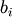are 1.

Parameters: x1, x2 : array_like Only integer types are handled (including booleans). out : array_like Result.

binary_repr
Return the binary representation of the input number as a string.

Examples

We’ve seen that 13 is represented by 00001101. Similary, 17 is represented by 00010001. The bit-wise AND of 13 and 17 is therefore 000000001, or 1:

>>> np.bitwise_and(13, 17)
1

>>> np.bitwise_and(14, 13)
12
>>> np.binary_repr(12)
'1100'
>>> np.bitwise_and([14,3], 13)
array([12,  1])

>>> np.bitwise_and([11,7], [4,25])
array([0, 1])
>>> np.bitwise_and(np.array([2,5,255]), np.array([3,14,16]))
array([ 2,  4, 16])
>>> np.bitwise_and([True, True], [False, True])
array([False,  True], dtype=bool)


#### Previous topic

Binary operations

numpy.bitwise_or